# Rotational Kinetic Energy Formula

Rotational Kinetic Energy Formula

Kinetic energy is the energy of moving objects, including objects that are rotating. The kinetic energy of a rotating object depends on the object's angular (rotational) velocity in radians per second, and on the object's moment of inertia. Moment of inertia is a measure of how easy it is to change the rotation of an object. Moments of inertia are represented with the letter I, and are expressed in units of kg∙m2. The unit of kinetic energy is Joules (J). In terms of other units, one Joule is equal to one kilogram meter squared per second squared (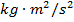).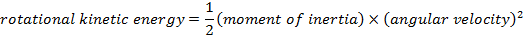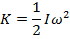K = kinetic energy (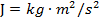)

I = moment of inertia (kg∙m2)

ω = angular velocity (radians/s)

Rotational Kinetic Energy Formula Questions:

1) A round mill stone with a moment of inertia of I = 1500 kg∙m2 is rotating at an angular velocity of 8.00 radians/s. What is the stone's rotational kinetic energy?

Answer: The rotational kinetic energy of the mill stone can be found using the formula: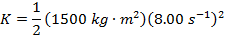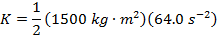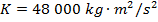K = 48 000 J

The rotational kinetic energy of the mill stone is 48 000 J.

2) What is the rotational kinetic energy of a DVD (digital video disc) with a moment of inertia of I = 1.000×10-4 kg∙m2, rotating at an angular velocity of 760.0 radians/s?

Answer: The rotational kinetic energy of the DVD can be found using the formula: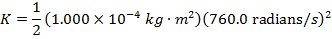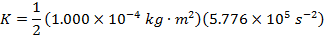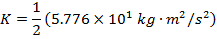K = 28.88 J

The kinetic energy of the rotating DVD is 28.88 J.